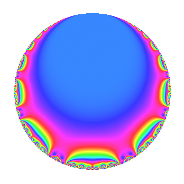Properties

 Label 100.2.aLevel 100 Weight 2 Character orbit a Rep. character $$\chi_{100}(1,\cdot)$$ Character field $$\Q$$ Dimension 1 Newforms 1 Sturm bound 30 Trace bound 0

Related objects

Defining parameters

 Level: $$N$$ = $$100 = 2^{2} \cdot 5^{2}$$ Weight: $$k$$ = $$2$$ Character orbit: $$[\chi]$$ = 100.a (trivial) Character field: $$\Q$$ Newforms: $$1$$ Sturm bound: $$30$$ Trace bound: $$0$$

Dimensions

The following table gives the dimensions of various subspaces of $$M_{2}(\Gamma_0(100))$$.

Total New Old
Modular forms 24 1 23
Cusp forms 7 1 6
Eisenstein series 17 0 17

The following table gives the dimensions of the cuspidal new subspaces with specified eigenvalues for the Atkin-Lehner operators and the Fricke involution.

$$2$$$$5$$FrickeDim.
$$-$$$$+$$$$-$$$$1$$
Plus space$$+$$$$0$$
Minus space$$-$$$$1$$

Trace form

 $$q$$ $$\mathstrut +\mathstrut 2q^{3}$$ $$\mathstrut -\mathstrut 2q^{7}$$ $$\mathstrut +\mathstrut q^{9}$$ $$\mathstrut +\mathstrut O(q^{10})$$ $$q$$ $$\mathstrut +\mathstrut 2q^{3}$$ $$\mathstrut -\mathstrut 2q^{7}$$ $$\mathstrut +\mathstrut q^{9}$$ $$\mathstrut -\mathstrut 2q^{13}$$ $$\mathstrut +\mathstrut 6q^{17}$$ $$\mathstrut -\mathstrut 4q^{19}$$ $$\mathstrut -\mathstrut 4q^{21}$$ $$\mathstrut -\mathstrut 6q^{23}$$ $$\mathstrut -\mathstrut 4q^{27}$$ $$\mathstrut +\mathstrut 6q^{29}$$ $$\mathstrut -\mathstrut 4q^{31}$$ $$\mathstrut -\mathstrut 2q^{37}$$ $$\mathstrut -\mathstrut 4q^{39}$$ $$\mathstrut +\mathstrut 6q^{41}$$ $$\mathstrut +\mathstrut 10q^{43}$$ $$\mathstrut +\mathstrut 6q^{47}$$ $$\mathstrut -\mathstrut 3q^{49}$$ $$\mathstrut +\mathstrut 12q^{51}$$ $$\mathstrut +\mathstrut 6q^{53}$$ $$\mathstrut -\mathstrut 8q^{57}$$ $$\mathstrut +\mathstrut 12q^{59}$$ $$\mathstrut +\mathstrut 2q^{61}$$ $$\mathstrut -\mathstrut 2q^{63}$$ $$\mathstrut -\mathstrut 2q^{67}$$ $$\mathstrut -\mathstrut 12q^{69}$$ $$\mathstrut -\mathstrut 12q^{71}$$ $$\mathstrut -\mathstrut 2q^{73}$$ $$\mathstrut +\mathstrut 8q^{79}$$ $$\mathstrut -\mathstrut 11q^{81}$$ $$\mathstrut -\mathstrut 6q^{83}$$ $$\mathstrut +\mathstrut 12q^{87}$$ $$\mathstrut -\mathstrut 6q^{89}$$ $$\mathstrut +\mathstrut 4q^{91}$$ $$\mathstrut -\mathstrut 8q^{93}$$ $$\mathstrut -\mathstrut 2q^{97}$$ $$\mathstrut +\mathstrut O(q^{100})$$

Decomposition of $$S_{2}^{\mathrm{new}}(\Gamma_0(100))$$ into irreducible Hecke orbits

Label Dim. $$A$$ Field CM Traces A-L signs $q$-expansion
$$a_2$$ $$a_3$$ $$a_5$$ $$a_7$$ 2 5
100.2.a.a $$1$$ $$0.799$$ $$\Q$$ None $$0$$ $$2$$ $$0$$ $$-2$$ $$-$$ $$+$$ $$q+2q^{3}-2q^{7}+q^{9}-2q^{13}+6q^{17}+\cdots$$

Decomposition of $$S_{2}^{\mathrm{old}}(\Gamma_0(100))$$ into lower level spaces

$$S_{2}^{\mathrm{old}}(\Gamma_0(100)) \cong$$ $$S_{2}^{\mathrm{new}}(\Gamma_0(20))$$$$^{\oplus 2}$$$$\oplus$$$$S_{2}^{\mathrm{new}}(\Gamma_0(50))$$$$^{\oplus 2}$$